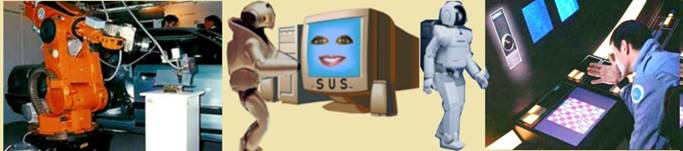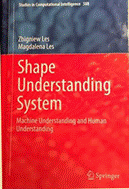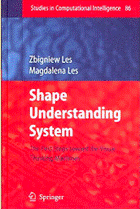SQJRIUMachine Understanding   Machine Understanding is the term introduced by Z. Les and M. Les to denote understanding by a machine.   Machine understanding is very different from artificial intelligence (AI) in terms of the assumptions, scientific methodology and terminology.   Machine understanding:   -       is the first attempt to establish the scientific method to investigate the complexity of understanding problem,   -       is based on the results of philosophical investigations and assumptions of the logical positivists,   -       is based on the development of the shape understanding system (SUS) and on the assumption that the results of understanding by the machine (SUS) can be evaluated according to the rules applied for evaluation of human understanding.   Machine understanding is defined as the problem solving and is focused on understanding  of categories of visual objects, categories of signs and the  categories of texts.   The most important part of machine understanding is evaluation of the result of understanding by formulating problems and testing if the machine is able to solve these problems. However, when the ability to solve problems can to some extent prove that machine has the ability to understand, the very important part of proving this is testing if the machine is able to explain how to solve these problems. However, even if machine will be able to explain how to solve the problems still there is a significant difference in machine understanding and human understanding.   Machine understanding refers to the categorical structure of learned knowledge and one of the most complex problems that is solved within this framework is understanding of visual objects (visual machine understanding).   Visual machine understanding is strictly connected with machine visual thinking and visual machine perception MU. Visual machine understanding is part of machine understanding concerning understanding of the visual object. The first stage of visual machine understanding involves perceptual reasoning that consists of the perceptual categorical reasoning and visual reasoning. The visual reasoning consists of assigned reasoning that assigns the perceived object to one of the shape categories. The assigned reasoning consists of the consecutive stages of reasoning where at each stage of reasoning the specific data are acquired based on the results of the reasoning at previous stages.       Last updated: 20 July 2019 Copyright the Queen Jadwiga Foundation Address: The Queen Jadwiga Foundation P.O. Box 654, Toorak, VIC 3142  Australia Machine Understanding